# Task 06: VAR & VECM¶

## Granger Causality¶

We say that $X$ Grager causes $Y$, if the past values of $X$ have explanatory power for current values of $Y$.

To test this, We will generate $X$ and $Y$ in the following way:
$$\begin{cases} X_t = 0.2 X_{t-1} + w_{t},\ w_t \sim \mathcal{N}(0,1) \\ Y_t = 0.3 Y_{t-1} - 0.5 Y_{t-2} + 0.2 Y_{t-3} - 0.2 X_{t-1} - 0.3 X_{t-2} - 0.1 X_{t-3} + \epsilon_t,\ \epsilon_t \sim \mathcal{N}(0,1) \end{cases}$$

In :
#Import the required modules for data generation
import numpy as np
#Import the required modules for plot creation:
import matplotlib.pyplot as plt
#Import the required modules for DataFrame creation:
import pandas as pd

#Import the required modules for TimeSeries data generation:
import statsmodels.api as sm
#Import the required modules for test statistic calculation:
import statsmodels.stats as sm_stat
#Import the required modules for model estimation:
import statsmodels.tsa as smt
#Import the required modules for optimization:
import scipy as scipy
import statsmodels.formula.api as smf


We will simulate for a sample size of 500:

In :
np.random.seed(1)

N = 500

X = np.zeros(N)
Y = np.zeros(N)

w = np.random.normal(loc = 0.0, scale = 1.0, size = N)
e = np.random.normal(loc = 0.0, scale = 1.0, size = N)


Generate $X$:

In :
X = w
for j in range(1, N):
X[j] = 0.2 * X[j - 1] + w[j]


Generate $Y$:

In :
Y = e
Y = 0.3 * Y - 0.2 * X + e
Y = 0.3 * Y - 0.5 * Y - 0.2 * X - 0.3 * X + e
for j in range(3, N):
Y[j] = 0.3 * Y[j - 1] - 0.5 * Y[j - 2] + 0.2 * Y[j - 3] - 0.2 * X[j - 1] - 0.3 * X[j - 2] - 0.1 * X[j - 3] + e[j]

In :
DT = pd.DataFrame([X, Y], index = ["X", "Y"]).T
DT.plot(subplots=True, layout=(2, 1))
plt.show()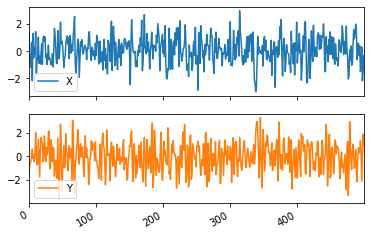### The Unrestricted model¶

We will begin by estimating an unrestricted model:

$$Y_t = \alpha + \delta t + \phi_1 Y_{t-1} + ... + \phi_p Y_{t-p} + \beta_1 X_{t-1} + ... + \beta_q X_{t-q} + \epsilon_t$$

We have already learned of the sequential procedure to choose $p$ and $q$ in the model. On the other hand, in the Granger causality case, this is not the question of the first importance and so, we will assume p = q.

In :
#Lag operator:
def L(x, n = 1):
if n == 0:
return x
if isinstance(x, pd.Series):
return x.shift(n)
else:
x = pd.Series(x)
return x.shift(n)

#Difference operator:
def d(x):
if isinstance(x, pd.Series):
return x.diff()
else:
x = pd.Series(x)
return x.diff()

#Trend/time operator:
def time(x):
return list(range(1, len(x) + 1))


We will also define a funtion to extract the coefficient table:

In :
#Return the model coefficients of a smf.ols(...).fit() object:
def get_coef(mdl_fit):
result = np.round(pd.DataFrame([mdl_fit.params, mdl_fit.tvalues, mdl_fit.pvalues], index = ["coef", "t-value", "p-value"]).T, 5)
return(result)


We will begin by setting $p=q=4$:

In :
mod_UR = smf.ols(formula = 'Y ~ 1 + time(Y) + L(Y, 1) + L(Y, 2) + L(Y, 3) + L(Y, 4) + L(X, 1) + L(X, 2) + L(X, 3) + L(X, 4)', data = DT).fit()
get_coef(mod_UR)

Out:
coef t-value p-value
Intercept 0.04786 0.54051 0.58909
time(Y) -0.00010 -0.31712 0.75129
L(Y, 1) 0.26118 5.81055 0.00000
L(Y, 2) -0.46762 -10.35969 0.00000
L(Y, 3) 0.16800 3.82247 0.00015
L(Y, 4) 0.03509 0.84809 0.39680
L(X, 1) -0.15171 -3.43602 0.00064
L(X, 2) -0.35091 -7.72272 0.00000
L(X, 3) -0.15685 -3.25712 0.00120
L(X, 4) 0.14562 3.00051 0.00283

We then remove L(Y, 4) and check for insignificant coefficients.

Then, we remove time(Y) (because it is insignificant).

While we know that there is no intercept in our data generating pricess (i.e. DGP), it is worth also checking the mean of the series:

In :
print("Series means are:\n", DT.mean(axis = 0))
print("Series variances are:\n", DT.var(axis = 0))

Series means are:
X    0.066959
Y   -0.016831
dtype: float64
Series variances are:
X    1.011222
Y    1.569013
dtype: float64


So, it appears that the series has a zero-mean. We can verify this via a t-test where the null hypothesis are $H_0: \mathbb{E}(Y_t) = 0$ and $H_0: \mathbb{E}(X_t) = 0$

In :
print(scipy.stats.ttest_1samp(DT["X"], popmean = 0))
print(scipy.stats.ttest_1samp(DT["Y"], popmean = 0))

Ttest_1sampResult(statistic=1.488923579649989, pvalue=0.13713920371635088)
Ttest_1sampResult(statistic=-0.30045795214060694, pvalue=0.7639530000751562)


So we do not reject the null hypothesis in either case since the p-value > 0.05 for both series. Because of this, and the fact that Intercept coefficient is also insignificant after removing the previous two coefficients - we remove the Intercept as well:

In :
mod_UR = smf.ols(formula = 'Y ~ -1 + L(Y, 1) + L(Y, 2) + L(Y, 3) + L(X, 1) + L(X, 2) + L(X, 3) + L(X, 4)', data = DT).fit()
get_coef(mod_UR)

Out:
coef t-value p-value
L(Y, 1) 0.26850 6.07557 0.00000
L(Y, 2) -0.48680 -12.57832 0.00000
L(Y, 3) 0.18030 4.34041 0.00002
L(X, 1) -0.14919 -3.39262 0.00075
L(X, 2) -0.34744 -7.68324 0.00000
L(X, 3) -0.15513 -3.23323 0.00131
L(X, 4) 0.14259 2.97370 0.00309

### The Restricted Model¶

Because we want to test, if $X_{t-j},\ j > 0$ improve the model of $Y_t$, we need to estimate a restricted model, where the restriction is that $\beta_1 = ... = \beta_q = 0$ :

$$Y_t = \alpha + \delta t + \phi_1 Y_{t-1} + ... + \phi_p Y_{t-p} + \epsilon_t$$

i.e. the coefficients of $X$ Series are insignificant.

In :
mod_R = smf.ols(formula = 'Y ~ -1 + L(Y, 1) + L(Y, 2) + L(Y, 3)', data = DT).fit()
get_coef(mod_R)

Out:
coef t-value p-value
L(Y, 1) 0.36183 8.20549 0.00000
L(Y, 2) -0.52855 -12.97914 0.00000
L(Y, 3) 0.19286 4.37158 0.00002

Note that we are not attempting to remove or add any coefficients to the Restricted model, as we are already imposing a restriction on $\beta_j$ coefficients, and we want to compare the $UR$ and $R$ models to see if those specific restrictions are valid.

### Model Comparison¶

To compare these two models, we will compare them by their SSR (sum of squared residuals, also known as residual sum of squares (RSS), or sum of squared errors of prediction (SSE)). We can do this by carrying out an ANOVA test.

In :
sm_stat.api.anova_lm(mod_R, mod_UR)

c:\users\andrius\appdata\local\programs\python\python38\lib\site-packages\scipy\stats\_distn_infrastructure.py:903: RuntimeWarning: invalid value encountered in greater
return (a < x) & (x < b)
c:\users\andrius\appdata\local\programs\python\python38\lib\site-packages\scipy\stats\_distn_infrastructure.py:903: RuntimeWarning: invalid value encountered in less
return (a < x) & (x < b)
c:\users\andrius\appdata\local\programs\python\python38\lib\site-packages\scipy\stats\_distn_infrastructure.py:1912: RuntimeWarning: invalid value encountered in less_equal
cond2 = cond0 & (x <= _a)

Out:
df_resid ssr df_diff ss_diff F Pr(>F)
0 494.0 561.093262 0.0 NaN NaN NaN
1 489.0 457.810248 5.0 103.283014 22.063898 5.886248e-20

Since p-value < 0.05, we reject the null hypothesis $H_0: SSR_R = SSR_{UR}$. This means that the models are not identical. To check, which model is better, we can look at the $SSR$ and the $AIC$, or $BIC$ values:

In :
pd.DataFrame([mod_R.ssr, mod_UR.ssr], index = ["Restricted", "Unrestricted"], columns = ["SSR"]).T

Out:
Restricted Unrestricted
SSR 561.093262 457.810248
In :
pd.DataFrame([mod_R.aic, mod_UR.aic], index = ["Restricted", "Unrestricted"], columns = ["AIC"]).T

Out:
Restricted Unrestricted
AIC 1476.709564 1381.846942
In :
pd.DataFrame([mod_R.bic, mod_UR.bic], index = ["Restricted", "Unrestricted"], columns = ["BIC"]).T

Out:
Restricted Unrestricted
BIC 1489.335334 1411.292974

We see that in all cases the $UR$ model is better: it has a lower SSR (i.e. the model errors are smaller) and a lower $AIC$ and $BIC$ value compares to the restricted model.

So, we can conclute that $X$ Granger causes $Y$.

Now we are interested if this is the case the other way around. Remembering that we set $p = q$, we are left with the following $UR$ model for $X$:

In :
X_UR = smf.ols(formula = 'X ~ -1 + L(Y, 1) + L(X, 1)', data = DT).fit()
get_coef(X_UR)

Out:
coef t-value p-value
L(Y, 1) 0.03078 0.87004 0.38470
L(X, 1) 0.18394 4.18541 0.00003

The restricted model:

In :
X_R = smf.ols(formula = 'X ~ -1 + L(X, 1)', data = DT).fit()
get_coef(X_R)

Out:
coef t-value p-value
L(X, 1) 0.18357 4.1781 0.00003

Now, we carry out the ANOVA test:

In :
sm_stat.api.anova_lm(X_R, X_UR)

Out:
df_resid ssr df_diff ss_diff F Pr(>F)
0 498.0 487.12786 0.0 NaN NaN NaN
1 497.0 486.38705 1.0 0.74081 0.756974 0.384697

Since p-value > 0.05, we do not reject the null hypothesis $H_0: SSR_R = SSR_{UR}$.

This means that the errors of the two models are simialr in magnitude. In other words, including the additional coefficients from the lags of $Y$ series does not improve our model for $X$, as our $SSR$ is not significantly different from the model $SSR$ without the lags of $Y$.

Note that the errors themselves are not identical, i.e. $e_{t, UR} \neq e_{t, R}$, the results say that overall their squared residual sums are not statistically significantly different.

In :
pd.DataFrame([X_R.ssr, X_UR.ssr], index = ["Restricted", "Unrestricted"], columns = ["SSR"]).T

Out:
Restricted Unrestricted
SSR 487.12786 486.38705
In :
pd.DataFrame([X_R.aic, X_UR.aic], index = ["Restricted", "Unrestricted"], columns = ["AIC"]).T

Out:
Restricted Unrestricted
AIC 1406.085005 1407.325562
In :
pd.DataFrame([X_R.bic, X_UR.bic], index = ["Restricted", "Unrestricted"], columns = ["BIC"]).T

Out:
Restricted Unrestricted
BIC 1410.297611 1415.750775

The $SSR$, $AIC$ and $BIC$ values are quite similar in both the $UR$ and $R$ cases.

We can conclude that $Y$ does not Granger cause $X$.

## VAR: Estimation and Forecasting¶

For the sake of this example, assume that our VAR model is of the form:

$$\begin{pmatrix}Y_{t} \\ X_{t} \end{pmatrix} = \begin{pmatrix} 3 \\ 0 \end{pmatrix} + \begin{pmatrix} 0.5 & 0.4 \\ -0.2 & 0.6 \end{pmatrix} \begin{pmatrix} Y_{t-1} \\ X_{t-1} \end{pmatrix} + \begin{pmatrix} -0.2 & -0.1 \\ 0.3 & 0.1 \end{pmatrix} \begin{pmatrix} Y_{t-2} \\ X_{t-2} \end{pmatrix} + \begin{pmatrix} \epsilon_{1,t} \\ \epsilon_{2,t} \end{pmatrix} ,\quad \begin{pmatrix} \epsilon_{1,t} \\ \epsilon_{2,t} \end{pmatrix} \sim \mathcal{N} \left( \begin{bmatrix} 0 \\ 0 \end{bmatrix} , \begin{bmatrix} 8 & 6 \\ 6 & 9 \end{bmatrix} \right)$$

i.e., we are analysing a $VAR(2)$ model with correlated residuals.

### Specifying the parameters¶

In :
nsample = 500

alpha = np.array([3, 0])
theta_1 = np.array([[0.5, 0.4], [-0.2, 0.6]])
theta_2 = np.array([[-0.2, -0.1], [0.3, 0.1]])

mu = [0, 0]
sigma = np.array([[8, 6],[6, 9]])


### Generating the residuals¶

Note that the covariance matrix is always both symmetric and positive semi-definite. Equivalently, its eigenvalues are non-negative. We can verify this:

In :
print("The eigenvalues: ", np.linalg.eigvals(sigma))
print("Are they non-negative? :", np.all(np.linalg.eigvals(sigma) >= 0))

The eigenvalues:  [ 2.47920271 14.52079729]
Are they non-negative? : True


We begin by generating our shocks, $\vec{\epsilon}_t$:

In :
np.random.seed(1)
e = np.random.multivariate_normal(mu, sigma, size = nsample)

In :
#Transform to DataFrame for easier ploting and stat calculation:
e_test = pd.DataFrame(e, columns = ["E1", "E2"])
e_test.plot(subplots=True, layout = (2, 1))
plt.show()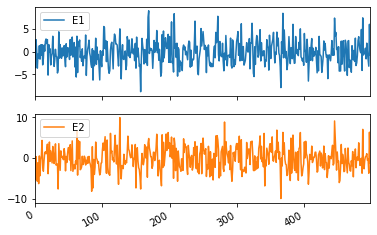In :
# The sample covariance:
e_test.cov()

Out:
E1 E2
E1 7.273977 5.685325
E2 5.685325 9.022872
In :
# The sample mean:
e_test.mean()

Out:
E1   -0.156420
E2   -0.098235
dtype: float64

### Generating the data¶

Now, we can generate the data:

In :
Y = np.zeros(shape = (nsample, 2))
#The first 5 rows:
Y[:5, :]

Out:
array([[0., 0.],
[0., 0.],
[0., 0.],
[0., 0.],
[0., 0.]])

When $t = 1$, we have:

In :
Y = alpha + e


When $t = 2$:

In :
Y = alpha + np.dot(theta_1, Y)  + e


When $t > 2$:

In :
for j in range(2, nsample):
Y[j] = alpha + np.dot(theta_1, Y[j - 1])  + np.dot(theta_2, Y[j - 2]) + e[j]


The resulting series:

In :
Y[:5, :]

Out:
array([[ -0.48231193,  -5.20715159],
[  3.28200428,  -2.69068434],
[  4.6157294 ,  -7.81673238],
[ -1.82613492, -10.60194712],
[ -2.82956403,  -6.55339816]])

Most of time series models in Python require that the pandas DataFrame object to have a valid date/time index, so we need to assign a date and frequency to the data - let's say that our data is daily, starting from 2014:

In :
Y = pd.DataFrame(Y, columns = ["Y", "X"])
Y.index = pd.date_range(start = "2014-01-01", periods = len(Y.index), freq = "D").to_period()
Y.index = Y.index.to_timestamp()

Out:
Y X
2014-01-01 -0.482312 -5.207152
2014-01-02 3.282004 -2.690684
2014-01-03 4.615729 -7.816732
2014-01-04 -1.826135 -10.601947
2014-01-05 -2.829564 -6.553398
In :
Y.plot(subplots=True, layout = (2, 1))
plt.show()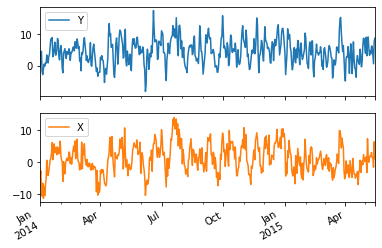### Checking if a specific VAR model has a unit root¶

We also want to see, if the series is $TS$ or $DS$. To do this, we can check if: $$\det (I - \Theta_1 z - \Theta_2 z^2 ) = 0 \iff |z_j | > 1,\ \forall j$$ i.e. if all the roots are outside the unit complex circle, then the process is stationary.

Fortunately, we can very easily do this in Python using symbolic expressions:

In :
from sympy import *

In :
z = Symbol('z')
D = Matrix([[1, 0], [0, 1]]) - Matrix(theta_1) * z - Matrix(theta_2) * (z**2)

In :
D

Out:
$\displaystyle \left[\begin{matrix}0.2 z^{2} - 0.5 z + 1 & 0.1 z^{2} - 0.4 z\\- 0.3 z^{2} + 0.2 z & - 0.1 z^{2} - 0.6 z + 1\end{matrix}\right]$

Next, we calculate the determinant:

In :
D.det()

Out:
$\displaystyle - \left(- 0.3 z^{2} + 0.2 z\right) \left(0.1 z^{2} - 0.4 z\right) + \left(- 0.1 z^{2} - 0.6 z + 1\right) \left(0.2 z^{2} - 0.5 z + 1\right)$

We can also multiply the expressions in parenthesis by converting the result into a into a polynomial object:

In :
temp_poly = Poly(D.det(), z)
temp_poly

Out:
$\displaystyle \operatorname{Poly}{\left( 0.01 z^{4} - 0.21 z^{3} + 0.48 z^{2} - 1.1 z + 1.0, z, domain=\mathbb{R} \right)}$
In :
temp_roots = roots(D.det(), z)
temp_roots

Out:
{1.23662943597335: 1,
18.7362676595998: 1,
0.513551452213425 - 2.01301370614087*I: 1,
0.513551452213425 + 2.01301370614087*I: 1}

The real solution is: \begin{aligned} z_1 &= 1.23662943597335\\ z_2 &= 18.7362676595998 \end{aligned} The complex solution is: \begin{aligned} z_3 &= 0.513551452213425 + 2.01301370614087 \cdot i\\ z_4 &= 0.513551452213425 - 2.01301370614087 \cdot i \end{aligned}

The additional values are their multiplicities. Also, more root calculation examples using python.

To calculate the absolute values, we need to extract the roots:

In :
temp_roots = [*temp_roots]
temp_roots

Out:
[1.23662943597335,
18.7362676595998,
0.513551452213425 - 2.01301370614087*I,
0.513551452213425 + 2.01301370614087*I]

And use the Abs() function from sympy. We also use N(expr, 4) function to round the sympy results (which are a sympy object and not an ordinary value) to 4 decimal precision:

In :
for j in temp_roots:
print("z = ", N(j, 4), "=> |z| = ", N(Abs(j), 4))

z =  1.237 => |z| =  1.237
z =  18.74 => |z| =  18.74
z =  0.5135 - 2.013*I => |z| =  2.077
z =  0.5135 + 2.013*I => |z| =  2.077


So, all the roots are outside the unit circle, which means that this $VAR$ model is stationary.

Note that in general we do not know the underlying DGP, so we would need to perform the $ADF$ or $KPSS$ tests on each variable to verify whether they have a unit root or not.

### Estimating a $VAR$ model¶

A VAR model can be stimated using the documented functions. It is worth reading the documentation as it not only provides examples on using the provided functions, but sometimes gives additional guidelines. For example:

The VAR class assumes that the passed time series are stationary.

Non-stationary or trending data can often be transformed to be stationary by first-differencing or some other method.

For direct analysis of non-stationary time series, a standard stable VAR(p) model is not appropriate.

In :
Y_VAR = smt.api.VAR(Y)


### Lag order selection¶

Choosing the lag order can be a difficult problem. Fortunately, we can use the automated procedure, which is based on calculating the information criteria for different lag orders:

Note: in case of errors like this one:

2-th leading minor of the array is not positive definite

make sure that the lag order is not too large (or the data sample too small). Also, this can happen if some roots $|z_j|$ are very close to 1 (but still greater than 1).

In :
Y_VAR.select_order(10)

Out:
<statsmodels.tsa.vector_ar.var_model.LagOrderResults at 0x1991c627820>

We see that the recommended model is $VAR(2)$ according to $AIC$, $BIC$, $HQIC$ and $FPE$ information criteria.

### Fitting the model¶

We will estimate a $VAR(2)$ model:

In :
results = Y_VAR.fit(2)
results.summary()

Out:
  Summary of Regression Results
==================================
Model:                         VAR
Method:                        OLS
Date:           Mon, 04, May, 2020
Time:                     23:50:18
--------------------------------------------------------------------
No. of Equations:         2.00000    BIC:                    3.61278
Nobs:                     498.000    HQIC:                   3.56141
Log likelihood:          -2281.79    FPE:                    34.0636
AIC:                      3.52823    Det(Omega_mle):         33.3897
--------------------------------------------------------------------
Results for equation Y
========================================================================
coefficient       std. error           t-stat            prob
------------------------------------------------------------------------
const         2.694126         0.241271           11.166           0.000
L1.Y          0.589969         0.050790           11.616           0.000
L1.X          0.370707         0.046172            8.029           0.000
L2.Y         -0.255685         0.049378           -5.178           0.000
L2.X         -0.075897         0.047882           -1.585           0.113
========================================================================

Results for equation X
========================================================================
coefficient       std. error           t-stat            prob
------------------------------------------------------------------------
const        -0.359460         0.268197           -1.340           0.180
L1.Y         -0.074297         0.056459           -1.316           0.188
L1.X          0.565941         0.051325           11.027           0.000
L2.Y          0.242305         0.054889            4.414           0.000
L2.X          0.099620         0.053225            1.872           0.061
========================================================================

Correlation matrix of residuals
Y         X
Y    1.000000  0.698762
X    0.698762  1.000000



We can also examine the error covariance matrix:

In :
results.resid.cov()

Out:
Y X
Y 7.208196 5.598939
X 5.598939 8.906885
In :
results.resid.mean()

Out:
Y   -5.243463e-16
X   -1.541382e-15
dtype: float64

The estimated model is: $$\begin{cases} \hat{Y}_t &= 2.694126 + 0.589969 \hat{Y}_{t-1} + 0.370707 \hat{X}_{t-1} -0.255685 \hat{Y}_{t-2} -0.075897 \hat{X}_{t-2} \\ \hat{X}_t &= -0.359460 -0.074297 \hat{Y}_{t-1} + 0.565941 \hat{X}_{t-1} + 0.242305 \hat{Y}_{t-2} + 0.099620 \hat{X}_{t-2} \end{cases}$$

The model residual covariance matrix is:

$$\hat{\Sigma}_\epsilon = \begin{bmatrix} 7.208196 & 5.598939 \\ 5.598939 & 8.906885 \end{bmatrix}$$

Compared to the true model:

$$\begin{pmatrix} Y_{t} \\ X_{t} \end{pmatrix} = \begin{pmatrix} 3 \\ 0 \end{pmatrix} + \begin{pmatrix} 0.5 & 0.4 \\ -0.2 & 0.6 \end{pmatrix} \begin{pmatrix} Y_{t-1} \\ X_{t-1} \end{pmatrix} + \begin{pmatrix} -0.2 & -0.1 \\ 0.3 & 0.1 \end{pmatrix} \begin{pmatrix} Y_{t-2} \\ X_{t-2} \end{pmatrix} + \begin{pmatrix} \epsilon_{1,t} \\ \epsilon_{2,t} \end{pmatrix} ,\quad \begin{pmatrix} \epsilon_{1,t} \\ \epsilon_{2,t} \end{pmatrix} \sim \mathcal{N} \left( \begin{bmatrix} 0 \\ 0 \end{bmatrix} , \begin{bmatrix} 8 & 6 \\ 6 & 9 \end{bmatrix} \right)$$

We see that, for the most part, the estimated parameters are close to the true parameter values. The same can be said for the error covariance matrix.

### Impulse-response functions¶

From the estimated VAR model, we can plot the impulse response functions of the endogenous variables.

Impulse responses are of interest in econometric studies: they are the estimated responses to a unit impulse in one of the variables. They are computed in practice using the $MA(\infty)$ representation of the $VAR(p$) process: $$\vec{Y}_t = \vec{\mu} + \sum_{i=0}^\infty \Phi_i \vec{\epsilon}_{t-i}, \quad \vec{\epsilon}_t \sim \mathcal{N}(\vec{0}, \hat{\Sigma}_\epsilon)$$ Orthogonalization is done using the Cholesky decomposition of the estimated error covariance matrix $\hat{\Sigma}_\epsilon$ and hence interpretations may change depending on variable ordering.

In :
irf = results.irf(15)


We can compare the standard deviation shock to the non-orthogonalized residuals:

In :
irf.plot(orth = False)

Out: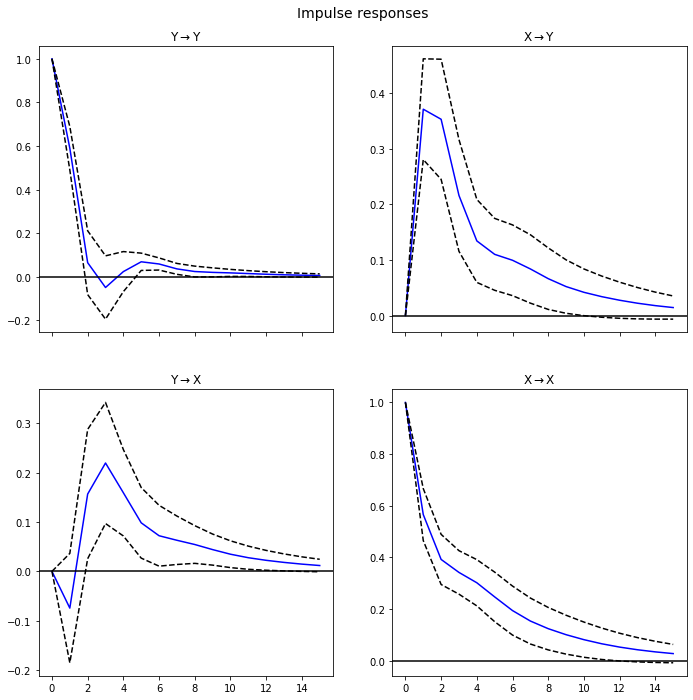To the unit shocks to the orthogonalized residuals:

In :
irf.plot(orth = True)

Out: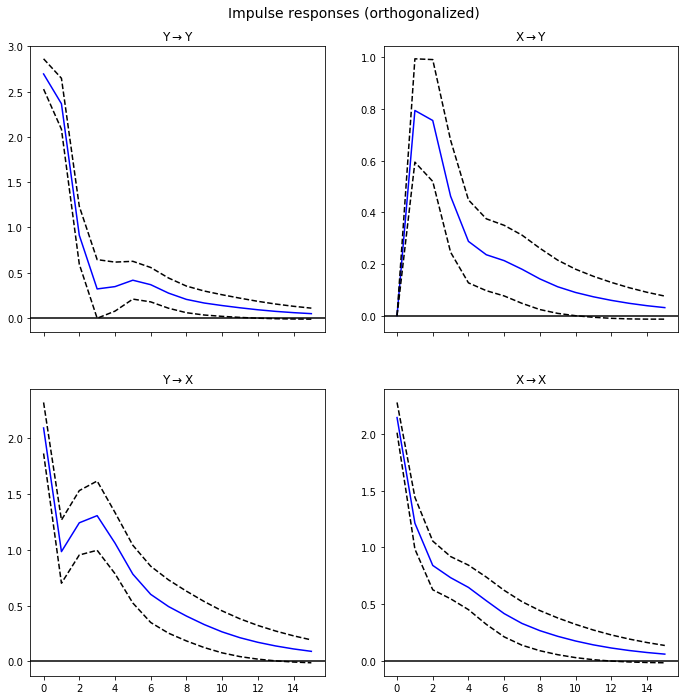Note that the main difference is the magnitude of the impulses and impulse of $Y$ and the response of $Y$ and $X$.

The cumulative effects $\Psi_n = \sum_{i=0}^n \Phi_i$ (i.e. where each period is the sum of the current and previous period response values) can be plotted with the long run effects:

In :
irf.plot_cum_effects(orth = False)

Out: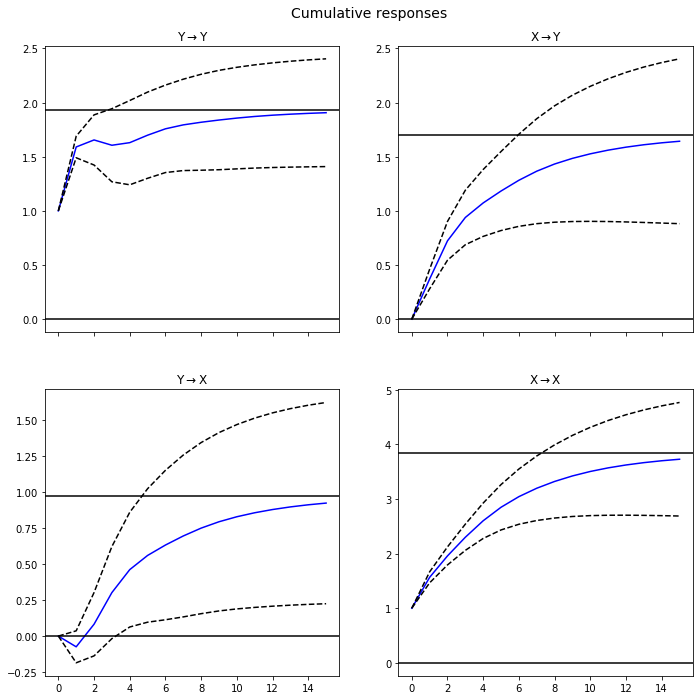### VAR model Forecasts¶

Finally, we cant calculate the forecasts:

In :
results.plot_forecast(10)
plt.xlim(xmin = 425) # we can subset the last variable forecast plot

Out:
(425, 534.45)